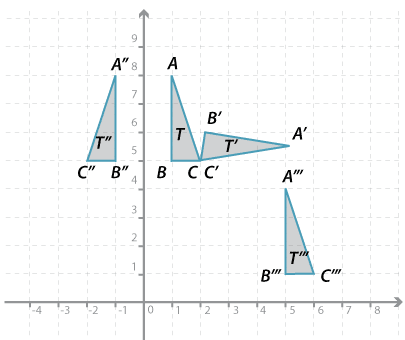# Year 7

## Measurement and Geometry

### Transformations of the planeDetailed description

Each of the triangles $$T^\prime$$, $$T^{\prime\prime}$$ and $$T^{\prime\prime\prime}$$ are obtained from triangle $$T$$ by moving it.

Triangle $$T^\prime$$ is formed by rotating triangle $$T$$ about the point $$C$$.

Triangle $$T^{\prime\prime}$$ is formed by reflecting triangle $$T$$ in the $$y$$-axis.

Triangle $$T^{\prime\prime\prime}$$ is formed by translating triangle $$T$$ across and down.

When the triangle is moved in this way we call the movement a transformation. The three types of transformations considered in this resource package are translations, reflections and rotations.

#### Properties of these transformations

When a figure is translated, rotated or reflected:

• Line segments move to intervals of the same length
• Angles move to angles of the same size.